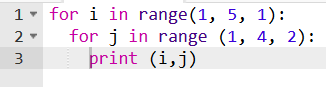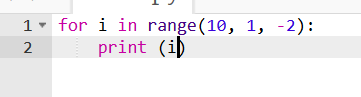Exercises to Practice (Module 7)

Lists and Other Data Structures

Exercise 1

1. Create a list containing three (3) breeds of dogs. Then use the “for” command to print this list.
2. Do exactly the same but use a tuple instead of a list.

Exercise 2

Ask the user for the name of the football team he or she supports. Print on the screen 10 times the answer.

Exercise 3

Ask the user for a number. Then create a program that will display the countdown from this number to 0.

Exercise 4

Create a program that reads 10 numbers from the keyboard and displays their average.

Exercise 5

Create a program that gets 10 names from the user, insert them in a list, and then print them out in the reverse order of the ones given.

Exercise 6

Create a program that will ask the user for a number and then print out a list of number from 1 to the number entered and the square of the number. For example, if the user entered '3' then the program would output:

1 squared is 1.

2 squared is 4.

3 squared is 9.

Exercise 7

What does the following section of the program prints out?Try writing the result on the paper, and then confirm by 'running' the code.

Exercise 8

How many times will the following “for” loop run?Try writing the result on the paper, and then confirm by 'running' the code.

Exercise 9

Create program that displays the odd numbers between 1 and 100.

Exercise 10

Create a program that calculates and prints out the sum of 1 + 2 + 3 + 4 .. + 100

ChallengeWrite a program that reads a 4-digit number from the keyboard and then prints out its digits in reverse order.

* Assume that the user always gives a 4-digit number.